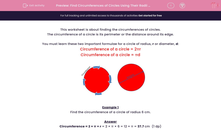# Find Circumferences of Circles Using Their Radii (2)

In this worksheet, students use formulae to calculate the circumferences of circles given their radii.This content is premium and exclusive to EdPlace subscribers.Key stage:  KS 2

Curriculum topic:   Maths and Numerical Reasoning

Curriculum subtopic:   2D Shapes: Circles, Angles and Bearings

Difficulty level:#### Worksheet Overview

This worksheet is about finding the circumferences of circles.

The circumference of a circle is its perimeter or the distance around its edge.

You must learn these two important formulae for a circle of radius, r or diameter, d:

Circumference of a circle = 2πr

Circumference of a circle = πdExample 1

Find the circumference of a circle of radius 6 cm.

Circumference = 2 × π × r = 2 × π × 6 = 12 × π = 37.7 cm  (1 dp)

Example 2

Find the circumference of a circle of diameter 6 cm.